﻿

### On the Relaxation of Some Types of Dirichlet Minimum Problems for Unbounded Functionals

#### Abstract

In this paper, considered a Borel function g on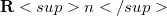$\mathbf {R}n$ taking its values in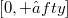$[0,+∈fty]$, verifying some weak hypothesis of continuity, such that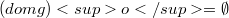$(domg)o = \emptyset$ and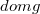$domg$ is convex, we obtain an integral representation result for the lower semicontinuous envelope in the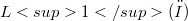$L1(ω)$ - topology of the integral functional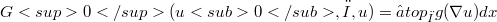$G0(u0,ω,u) = ∈top \limit _{ω}g(\nabla u)dx$, where(Error rendering LaTeX formula) only on suitable pin is of the boundary of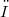$ω$ that lie, for example, on affine spaces orthogonal to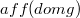$aff(domg)$, for boundary values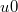$u{0}$ satisfying suitable compatibility conditions and$ω$ is geometrically well situated respect to$domg$. Then we apply this result to Dirichlet nunimum problems.

DOI Code: 10.1285/i15900932v19n2p231

Classification: 49J45

Full Text: PDF